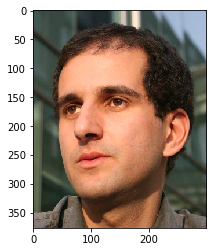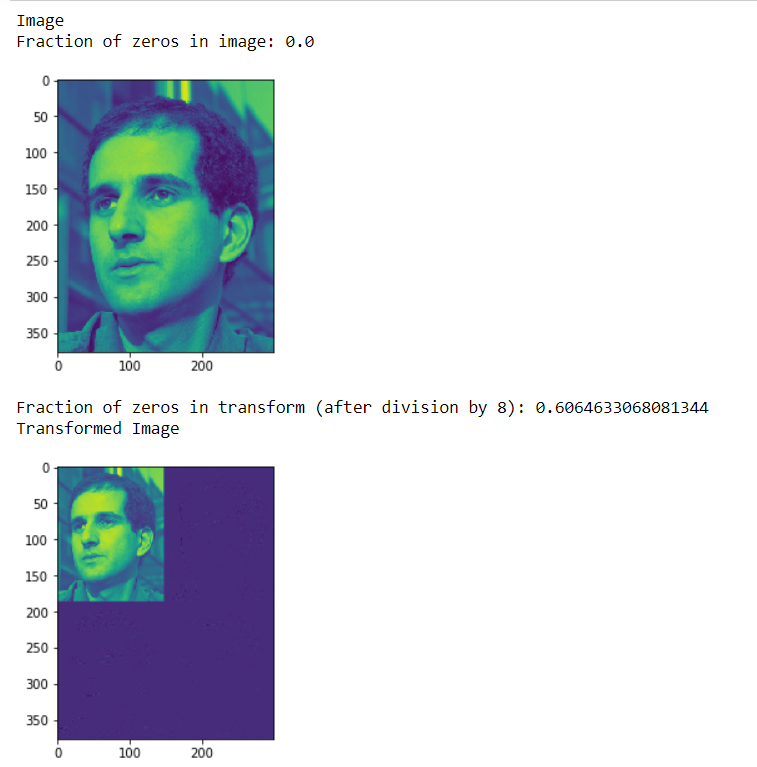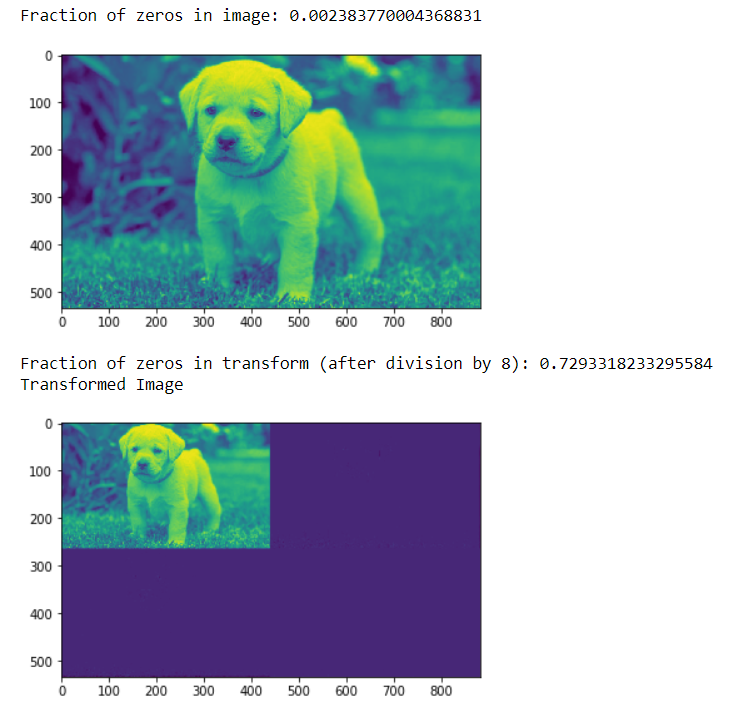Related Articles

# Mahotas – Fraction of zeros in image

• Last Updated : 15 Apr, 2021

In this article we will see how we can get the fraction of zeros in the image in mahotas. Fraction of zeros is the percentage amount of statistical data which is zero. It is relevant in statistical models where a significant amount of objects has zero value.
In this tutorial we will use “luispedro” image, below is the command to load it.

`mahotas.demos.load('luispedro')`

Below is the luispedro imageIn order to do this we will use np.mean method

Syntax : np.mean(img==0)
Argument : It takes image object as argument
Return : It returns numpy.float64

Note : Input image should be filtered or should be loaded as grey
In order to filter the image we will take the image object which is numpy.ndarray and filter it with the help of indexing, below is the command to do this

`image = image[:, :, 0]`

Example 1:

## Python3

 `# importing various libraries``import` `numpy as np``import` `mahotas``import` `mahotas.demos``from` `mahotas.thresholding ``import` `soft_threshold``from` `pylab ``import` `imshow, show``from` `os ``import` `path` `# loading image``f ``=` `mahotas.demos.load(``'luispedro'``, as_grey ``=` `True``)`  `# showing image``print``(``"Image"``)` `# getting fraction of zeros in image``fraction ``=` `np.mean(f ``=``=` `0``)` `print``(``"Fraction of zeros in image: {0}"``.``format``(fraction))``imshow(f)``show()`   `# Transform using D8 Wavelet to obtain transformed image t``t ``=` `mahotas.daubechies(f, ``'D8'``)` `# Discard low-order bits:``t ``/``=` `8``t ``=` `t.astype(np.int8)`  `# getting fraction of zeros in image``fraction ``=` `np.mean(t ``=``=` `0``)` `print``(``"Fraction of zeros in transform (after division by 8): {0}"``.``format``(fraction))` `# showing transformed image``print``(``"Transformed Image"``)``imshow(t)``show()`

Output :Example 2:

## Python3

 `# importing required libraries``import` `mahotas``import` `numpy as np``from` `pylab ``import` `imshow, show``import` `os`  `# loading image``img ``=` `mahotas.imread(``'dog_image.png'``)` `# filtering image``img ``=` `img[:, :, ``0``]`  `# getting fraction of zeros in image``fraction ``=` `np.mean(img ``=``=` `0``)` `print``(``"Fraction of zeros in image: {0}"``.``format``(fraction))``imshow(img)``show()`   `# Transform using D8 Wavelet to obtain transformed image t``t ``=` `mahotas.daubechies(img, ``'D8'``)` `# Discard low-order bits:``t ``/``=` `8``t ``=` `t.astype(np.int8)`  `# getting fraction of zeros in image``fraction ``=` `np.mean(t ``=``=` `0``)` `print``(``"Fraction of zeros in transform (after division by 8): {0}"``.``format``(fraction))` `# showing transformed image``print``(``"Transformed Image"``)``imshow(t)``show()`

Output :Attention geek! Strengthen your foundations with the Python Programming Foundation Course and learn the basics.

To begin with, your interview preparations Enhance your Data Structures concepts with the Python DS Course. And to begin with your Machine Learning Journey, join the Machine Learning – Basic Level Course

My Personal Notes arrow_drop_up